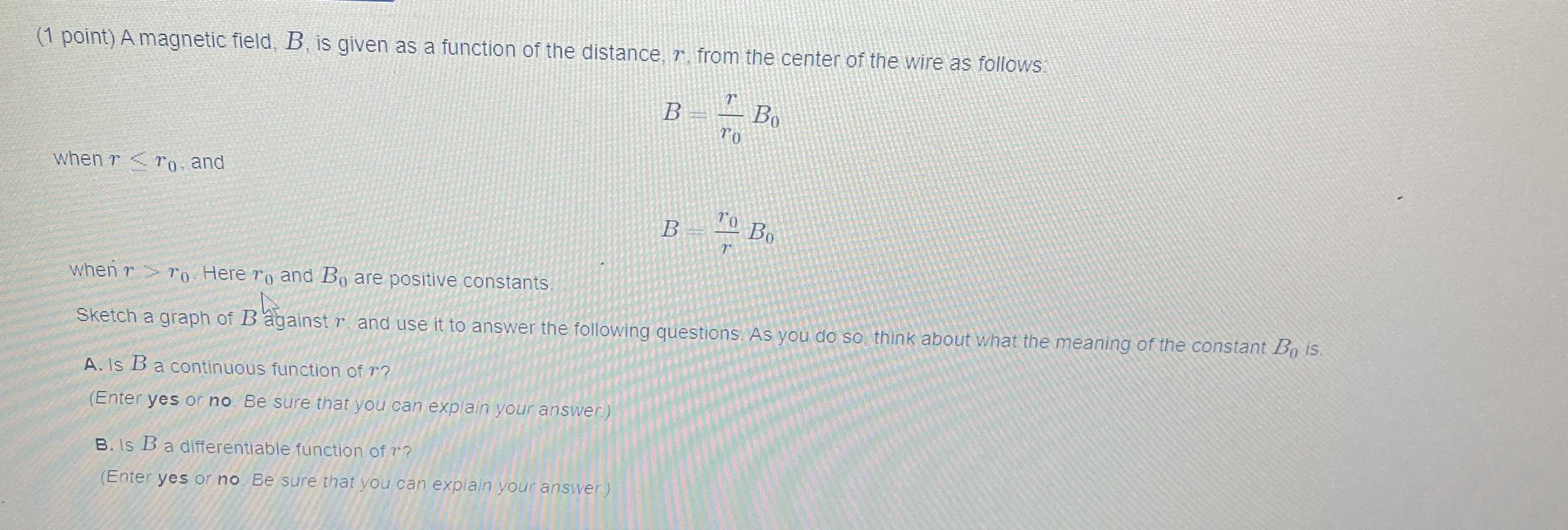### ¿Todavía tienes preguntas de matemáticas?

Pregunte a nuestros tutores expertos
Algebra
Pregunta(1 point) A magnetic field, $$B$$ , is given as a function of the distance, $$r$$ , from the center of the wire as follows: when $$r \leq r _ { 0 }$$ , and $$B = \frac { r } { r _ { 0 } } B _ { 0 }$$

Wheri $$r > r _ { 0 }$$ . Here $$r _ { 0 }$$ and $$B _ { 0 }$$ are positive constants. Sketch a graph of $$B$$ against $$r$$ and use it to answer the following questions. As you do so, think about what the meaning of the constant $$B _ { 0 }$$ is. A. Is $$B$$ a continuous function of $$r$$ ? (Enter yes or no Be sure that you can explain your answer.) B. Is $$B$$ a differentiable function of $$r$$ ? (Enter yes or no Be sure that you can explain your answer)